NCERT Solutions: Polynomials (Exercise 2.3)

# Polynomials (Exercise 2.3) NCERT Solutions - Class 10 Mathematics by VP Classes

EXERCISE 2.3
Ques 1: Divide the polynomial p(x) by the polynomial g(x) and find the quotient and remainder in each of the following :
(i) p(x) = x3 - 3x2 + 5x - 3, g(x) = x2 - 2

(ii) p(x) = x4 - 3x2 + 4x + 5, g(x) = x2 + 1 - x
(iii) p(x) = x4 - 5x + 6, g(x) = 2 - x2
Sol: Here, dividend p(x) = x3 - 3x2 + 5x - 3
divisor g(x) = x2 - 2
∴ We have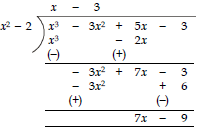Thus, the quotient = (x - 3) and remainder = (7x - 9)

(ii) Here, dividend p(x) = x- 3x2 + 4x + 5
and divisor g(x) = x2 + 1 - x
= x2 - x + 1
∴ We have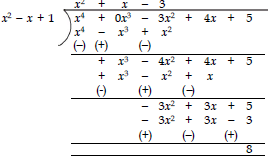Thus, the quotient is (x2 + x - 3) and remainder = 8

(iii) Here, dividend, p(x) = x4 - 5x + 6
and divisor, g(x) = 2 - x2
= - x2 + 2
∴ We have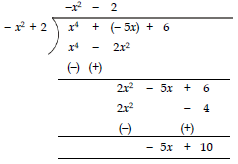Thus, the quotient = –x2 – 2 and remainder = –5x + 10.

Ques 2: Check whether the first polynomial is a factor of the second polynomial by dividing the second polynomial by the first polynomial: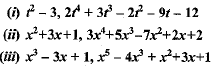Sol. (i) Dividing 2t4 + 3t3 - 2t2 - 9t - 12 by t2 - 3, we have: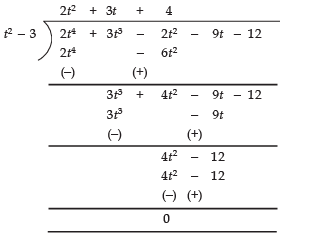∵ Remainder = 0
∴ (t2 - 3) is a factor of 2t4 + 3t3 - 2t2 - 9t - 12.

(ii) Dividing 3x4 + 5x3 - 7x2 + 2x + 2 by x2 + 3x + 1, we have: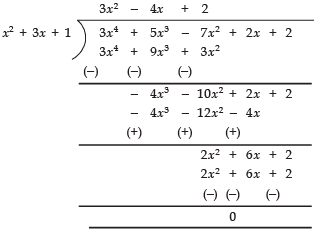∵ Remainder = 0.
∴ x2 + 3x + 1 is a factor of 3x4 + 5x3 - 7x+ 2x + 2.

(iii) Dividing x5 - 4x3 + x2 + 3x + 1 by x3 - 3x + 1, we get: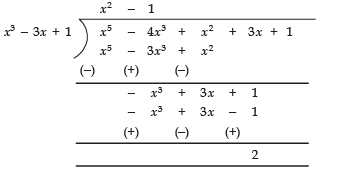∴ The remainder = 2, i.e., remainder ≠ 0
∴ x3 - 3x + 1 is not a factor of x- 4x3 + x2 + 3x + 1.

Ques 3: Obtain all other zeroes of 3x4 + 6x3 - 2x2 - 10x - 5, if two of its zeroes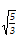are -.
Sol: We have p(x) = 3x4 + 6x- 2x2 - 10x - 5
α =and β = -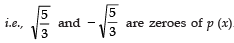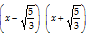is a factor of p (x).

Now, let us divide 3x4 + 6x3 - 2x2 - 10x - 5 by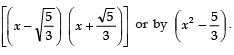.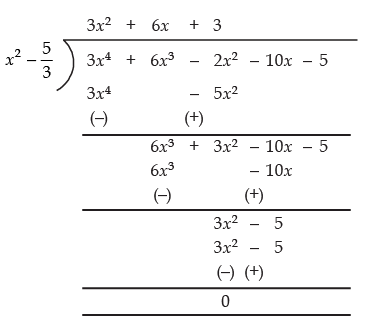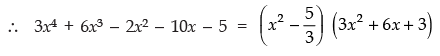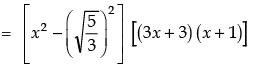For p (x) = 0, we have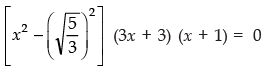i.e.,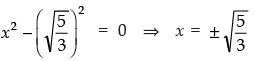or 3x + 3 = 0 ⇒ x = - 1
or x + 1 = 0 ⇒ x = - 1
Thus, all the other zeroes of the given polynomial are - 1 and - 1.

Ques 4: On dividing x- 3x2 + x + 2 by a polynomial g(x), the quotient and remainder were x - 2 and -2x + 4 respectively. Find g(x).
Sol: Here,
Dividend p(x) = x3 - 3x2 + x + 2
Divisor = g(x)
Quotient = (x - 2)
Remainder = (-2x + 4)
Since,

(Quotient × Divisor) + Remainder = Dividend

∴ [(x − 2) × g(x)] + [(−2x + 4)] = x3 − 3x2 + x + 2
⇒ (x − 2) × g(x)= x3 − 3x2 + x + 2 − (−2x + 4)
= x3 − 3x2 + x + 2 + 2x −4

= x3 - 3x2 + 3x - 2
∴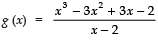Now, dividing x3 - 3x+ 3x - 2 by x - 2, we have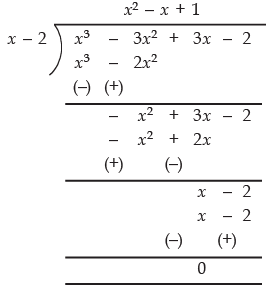∴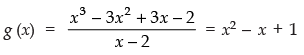Thus, the required divisor g(x) = x- x + 1.

Ques 5: Give example of polynomials p(x), g(x), q(x) and r(x), which satisfy the division algorithm and
(i) deg p(x) = deg q(x)
(ii) deg q(x) = deg r(x)
(iii) deg r(x) = 0

Sol:
(i) p(x),  g(x), q(x), r(x)
deg p(x) = deg q(x)
∴ both g(x) and r(x) are constant terms.
p(x) = 2x2- 2x + 14
g(x) = 2
q(x) = x2 - x + 7
r(x) = 0

(ii) deg q(x) = deg r(x)
∴ this is possible when deg of both q(x) and r(x) should be less than p(x) and g(x).
p(x) = x3 + x2 + x + 1
g(x) = x2 - 1
q(x) = x + 1, r(x) = 2x + 2
(iii) deg r(x) is 0.
This is possible when product of q(x) and g(x) form a polynomial whose degree is equal to degree of p(x) and constant term.

NOTE: We have given one example for each of the above cases, however, there can be several examples for them.

The document Polynomials (Exercise 2.3) NCERT Solutions | Class 10 Mathematics by VP Classes is a part of the Class 10 Course Class 10 Mathematics by VP Classes.
All you need of Class 10 at this link: Class 10

132 docs

## FAQs on Polynomials (Exercise 2.3) NCERT Solutions - Class 10 Mathematics by VP Classes

 1. What is a polynomial?Ans. A polynomial is an algebraic expression consisting of variables, coefficients, and exponents, combined using addition, subtraction, and multiplication operations. It can have one or more terms, and each term can have a different degree.
 2. How do you identify the degree of a polynomial?Ans. To identify the degree of a polynomial, you need to find the highest power of the variable in the polynomial. For example, in the polynomial 3x^2 + 5x - 2, the degree is 2 because the highest power of x is 2.
 3. What is the difference between a monomial and a binomial?Ans. A monomial is a polynomial with only one term, while a binomial is a polynomial with exactly two terms. For example, 3x^2 is a monomial, and 3x^2 + 5x is a binomial.
 4. How do you add or subtract polynomials?Ans. To add or subtract polynomials, you simply combine like terms. Like terms have the same variable(s) raised to the same power(s). Add the coefficients of the like terms while keeping the variable(s) and exponent(s) unchanged.
 5. Can a polynomial have a negative degree?Ans. No, a polynomial cannot have a negative degree. The degree of a polynomial is always a non-negative integer. The degree represents the highest power of the variable in the polynomial, and negative powers are not allowed in polynomials.

## Class 10 Mathematics by VP Classes

132 docsExplore Courses for Class 10 examSignup to see your scores go up within 7 days! Learn & Practice with 1000+ FREE Notes, Videos & Tests.
10M+ students study on EduRev
Track your progress, build streaks, highlight & save important lessons and more!
Related Searches

,

,

,

,

,

,

,

,

,

,

,

,

,

,

,

,

,

,

,

,

,

;